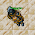## Wednesday, December 15, 2021

### TibiaGoals Riddles E14R08

Comment down below!

1.6 + 9 = 15
Toczek First

2.Happy Wednesday! :) I hope you're doing well.
I'd take the upper-right arrow of the 19 and move it to the lower-left of the 5.
The equation now says "6 + 9 = 15". :) Cheers! -Elyrea

3.Tamarizz / Solidera

Move one arrow from the 19 to make it a 13, into the 9 to make it an 8.

so it would be: 5 + 8 = 13

4.5.6+9=15
tris merigold

6.5+8=13

Shizurie

7.By moving the upper right arrow of the number 9 to bottom left of the number 5 the equation becomes 6+9=15

-Essiee

8.6 + 9 = 15
Character Name: Dyaniixz

9.6 + 9 = 15
Character Name: Dyaniixz

10.the number 5 turn it into 6, number 9 stays the same, number 19 turn it into 15
6+9=15
character name: pablosaurio

11.the number 5 turn it into 6, number 9 stays the same, number 19 turn it into 15
6+9=15
character name: pablosaurio

12.the number 5 turn it into 6, number 9 stays the same, number 19 turn it into 15
6+9=15
character name: pablosaurio

13.5 + 8 = 13
Amy Meow

14.15.16.17.18.19.6 + 9 = 15
Mian Stone'arrow

20.5+8=13; Lord Neganek

21.22.23.Answer: 6+9=15 (We have to move top-right arrow from'19 and put it in the bottom left on'5)
Kenlux, Antica

24.5+8=13 Mikolaj

25.5 + 8 = 13
righo :)

26.5 + 8 = 13 /Norelli

27.5+8=13

Xarkost Eilia

28.Psykeeh

5 + 8 = 13

29.30.5+8=13

Skyelie Hasulhoff <3

31.6+9 = 15

Toxic Hasulhoff

32.Congratulations to all participants!

You can control your results in our sheet: Riddles / Information

Remember, even if you got disqualified from the lottery, you can still participate in TibiaGoals riddles just for fun!

See you on Sunday!